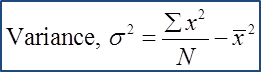# 8.2.4 Variance and Standard Deviation

Variance and Standard Deviation

1. The variance is a measure of the mean for the square of the deviations from the mean.

2. The standard deviation refers to the square root for the variance.

(A) Ungrouped DataExample 1:
Find the variance and standard deviation of the following data.
15, 17, 21, 24 and 31

Solution: